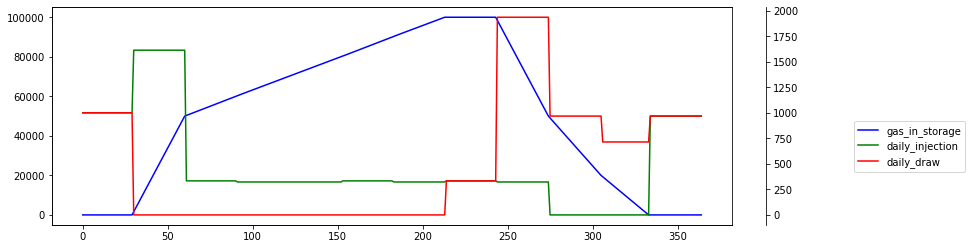In :
import matplotlib.pyplot as plt
import pandas as pd
import pulp

In :
curve = [
"Apr'21", 2.921,
"May'21", 2.892,
"Jun'21", 2.922,
"Jul'21", 2.96,
"Aug'21", 2.974,
"Sep'21", 2.966,
"Oct'21", 3.007,
"Nov'21", 3.07,
"Dec'21", 3.203,
"Jan'22", 3.294,
"Feb'22", 3.216,
"Mar'22", 3.03,
"Apr'22", 2.568,
"May'22", 2.48,
"Jun'22", 2.525,
"Jul'22", 2.562,
"Aug'22", 2.57,
"Sep'22", 2.559,
"Oct'22", 2.592,
"Nov'22", 2.681,
"Dec'22", 2.87,
"Jan'23", 2.987,
"Feb'23", 2.939,
"Mar'23", 2.779,
"Apr'23", 2.388
]

In :
df = pd.DataFrame( data={ 'Contract': curve[0::2], 'Price': curve[1::2]})


Forward curve exhibits strong seasonality

In :
df.plot(x='Contract', y='Price')

Out:
<AxesSubplot:xlabel='Contract'>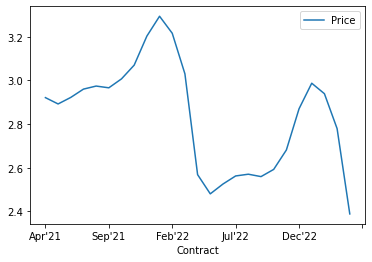Storage contract parameters

In :
N=12 # number of months to use
max_storage_capacity = 100000
max_daily_injection = pd.DataFrame(data=[(0.0,2000),(0.5,2000),(1.1,2000)], columns=['ratchet', 'mdiq'] )
max_daily_withdrawal = pd.DataFrame( data=[(0.0,4000),(0.5,4000),(1.1,4000)], columns=['ratchet', 'mdwq'] )

In :
# injection / withdrawal quantities
I=[None]*N
W=[None]*N
for i in range(N):
I[i] = pulp.LpVariable(f'I_{i}', lowBound=0, cat='Integer')
W[i] = pulp.LpVariable(f'W_{i}', lowBound=0, cat='Integer')

In :
# number of days in each injection / drawing month
days = list( map( lambda x: ((x+pd.DateOffset(months=1))-x).days, df.Contract.apply( lambda x: pd.to_datetime( '1-' + x ))))

# number of calendar days in the simulation
K = sum( days[0:N])

prices = list(df.Price)


This is main problem formulation. It is split by injection and withdrawal operation

In :
injection_charges=[None]*N
total_injection=[None]*N
daily_injection=[None]*K

# for each injection month
for i in range(N):
total_injection[i] = I[i]*10000
injection_charges[i] = -total_injection[i] * prices[i]

for i in range(N):
lb = sum(days[0:i])
ub = sum(days[:i+1])
daily_injection[lb:ub] = [ total_injection[i] / days[i] ] * days[i]

withdrawal_credit=[None]*N
total_draw=[None]*N
daily_draw=[None]*K

# for each drawing month
for j in range(N):
total_draw[j] = W[j]*10000
withdrawal_credit[j] = total_draw[j] * prices[j]

for i in range(N):
lb = sum(days[0:i])
ub = sum(days[:i+1])
daily_draw[lb:ub]  = [ total_draw[i] / days[i] ] * days[i]

daily_storage=[None]*K
for i in range(K):
if i == 0:
daily_storage[i] = daily_injection[i] - daily_draw[i]
else:
daily_storage[i] = daily_injection[i] - daily_draw[i] + daily_storage[i-1]


Here we are defining set of binary variables that will help to partition ratchets

In :
# ratchet range control variables
irc = [[None] * 2 for _ in range(K-1)]
wrc = [[None] * 2 for _ in range(K-1)]

for i in range(0,K-1):
for j in range(max_daily_injection.shape-1):
irc[i][j] = pulp.LpVariable(f'ic_{i+1}_{j}', cat='Binary')

for j in range(max_daily_withdrawal.shape-1):
wrc[i][j] = pulp.LpVariable(f'wc_{i+1}_{j}', cat='Binary')


Build_model is a function that defines constraints. The trick here is to use big M technique

In :
def build_model( max_daily_injection, max_daily_withdrawal):
m = pulp.LpProblem("WithRatchetsDaily", pulp.LpMaximize)

# target function
m += pulp.lpSum(withdrawal_credit) + pulp.lpSum(injection_charges)

for i in range(K):
m += daily_storage[i] >= 0
m += daily_storage[i] <= max_storage_capacity

# storage is empty on day 0
m += daily_injection <= max_daily_injection.loc[0, 'mdiq']
m += daily_draw <= max_daily_withdrawal.loc[ 0, 'mdwq']

M1 = -200 # lower boundary for the ratchet range
M2 = 200  # higher boundary for the ratchet range

# ratchet control
for i in range(K-1):

# injection
# only one range is allowed
m += pulp.lpSum( irc[i] ) == 1

# first range
m += irc[i] * max_daily_injection.loc[0,'ratchet'] + (1 - irc[i] ) * M1 \
<=  daily_storage[i] / max_storage_capacity
m += irc[i] * max_daily_injection.loc[1,'ratchet'] + (1 - irc[i] ) * M2 \
>=  daily_storage[i] / max_storage_capacity

# second range
m += irc[i] * max_daily_injection.loc[1,'ratchet'] + (1 - irc[i] ) * M1 \
<=  daily_storage[i] / max_storage_capacity
m += irc[i] * max_daily_injection.loc[2,'ratchet'] + (1 - irc[i] ) * M2 \
>=  daily_storage[i] / max_storage_capacity

# daily rate constraint
m += daily_injection[i+1] <= irc[i] * max_daily_injection.loc[1,'mdiq'] + \
irc[i] * max_daily_injection.loc[2,'mdiq']

# withdrawal
# only one range is allowed
m += pulp.lpSum( wrc[i] ) == 1

# first range
m += wrc[i] * max_daily_withdrawal.loc[0,'ratchet'] + ( 1 - wrc[i] ) * M1 \
<=  daily_storage[i] / max_storage_capacity
m += wrc[i] * max_daily_withdrawal.loc[1,'ratchet'] + ( 1 - wrc[i]) * M2 \
>= daily_storage[i] / max_storage_capacity

# second range
m += wrc[i] * max_daily_withdrawal.loc[1,'ratchet'] + ( 1 - wrc[i] ) * M1 \
<=  daily_storage[i] / max_storage_capacity
m += wrc[i] * max_daily_withdrawal.loc[2,'ratchet'] + ( 1 - wrc[i]) * M2 \
>= daily_storage[i] / max_storage_capacity

# daily rate constraint
m += daily_draw[i+1] <= wrc[i] * max_daily_withdrawal.loc[1,'mdwq'] + \
wrc[i] * max_daily_withdrawal.loc[2,'mdwq']

# ensure that storage is empty on the last day
m += daily_storage[K-1] == 0

return m


Baseline scenario. Max daily injection quantity is flat at 2000 Dth per day

In :
m = build_model( max_daily_injection, max_daily_withdrawal )
solver = pulp.getSolver('PULP_CBC_CMD')
m.solve(solver)
pulp.LpStatus[m.status], pulp.value(m.objective)

Out:
('Optimal', 39519.99999999997)

The following cell shows required hedges. For example we need to buy 4 April'2021 contracts and sell 12 Sep'2021 contracts

In :
for variable in m.variables():
if variable.varValue != 0.0 and variable.name[:2] in ('I_', 'W_'):
print( "{} = {}".format(variable.name, variable.varValue) )

I_0 = 4.0
I_1 = 6.0
I_10 = 5.0
I_3 = 6.0
I_4 = 6.0
I_5 = 6.0
I_6 = 6.0
I_8 = 6.0
W_10 = 5.0
W_3 = 6.0
W_4 = 12.0
W_6 = 6.0
W_8 = 6.0
W_9 = 10.0

In :
df_result = pd.DataFrame( data={
'gas_in_storage': map(lambda x: pulp.value(x) , daily_storage),
'daily_injection': map(lambda x: pulp.value(x), daily_injection),
'daily_draw': map(lambda x: pulp.value(x), daily_draw),
})

fig, ax = plt.subplots()
ax3 = ax.twinx()
rspine = ax3.spines['right']
rspine.set_position(('axes', 1.05))
ax3.set_frame_on(True)
ax3.patch.set_visible(False)

df_result['gas_in_storage'].plot(ax=ax, style='b-')
df_result['daily_injection'].plot(ax=ax3, style='g-')
df_result['daily_draw'].plot(ax=ax3, style='r-')

ax3.legend([ax.get_lines(), ax3.get_lines(), ax3.get_lines()], ['gas_in_storage','daily_injection', 'daily_draw'], bbox_to_anchor=(1.35, 0.5))

Out:
<matplotlib.legend.Legend at 0x7f56a5834cc0>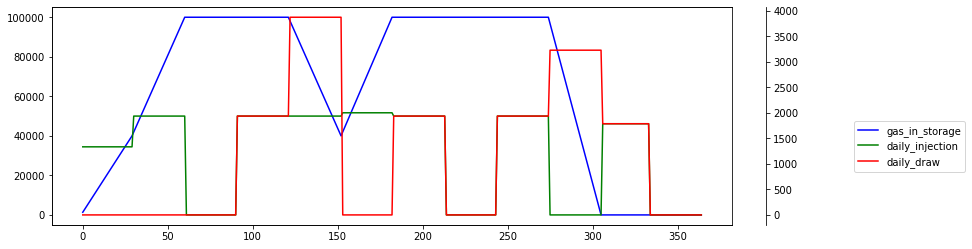We're going to run simulation when injection ratchet is reduced to 1000 Dth per day at 50% storage level. Note that profit now is lower by 11% vs flat ratchet scenario

In :
max_daily_injection2 = pd.DataFrame(data=[(0.0,2000),(0.5,2000),(1.1,1000)], columns=['ratchet', 'mdiq'] )
max_daily_withdrawal2 = pd.DataFrame( data=[(0.0,2000),(0.5,2000),(1.1,4000)], columns=['ratchet', 'mdwq'] )

m = build_model( max_daily_injection2, max_daily_withdrawal2 )
solver = pulp.getSolver('PULP_CBC_CMD')
m.solve(solver)
pulp.LpStatus[m.status], pulp.value(m.objective)

Out:
('Optimal', 35269.999999999985)

Injection and withdrawal ratchets look like this now

In :
max_daily_injection2.plot(x='ratchet',y='mdiq', style='g-', drawstyle="steps" )
max_daily_withdrawal2.plot(x='ratchet',y='mdwq',style='b-', drawstyle="steps")

Out:
<AxesSubplot:xlabel='ratchet'>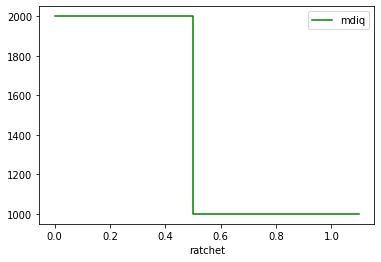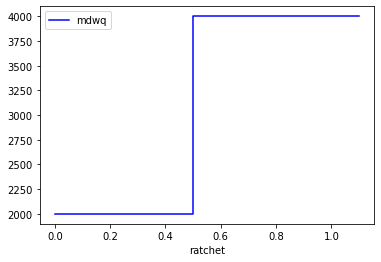In :
df_result = pd.DataFrame( data={
'gas_in_storage': map(lambda x: pulp.value(x) , daily_storage),
'daily_injection': map(lambda x: pulp.value(x), daily_injection),
'daily_draw': map(lambda x: pulp.value(x), daily_draw),
})

fig, ax = plt.subplots()
ax3 = ax.twinx()
rspine = ax3.spines['right']
rspine.set_position(('axes', 1.05))
ax3.set_frame_on(True)
ax3.patch.set_visible(False)

df_result['gas_in_storage'].plot(ax=ax, style='b-')
df_result['daily_injection'].plot(ax=ax3, style='g-')
df_result['daily_draw'].plot(ax=ax3, style='r-')

ax3.legend([ax.get_lines(), ax3.get_lines(), ax3.get_lines()], ['gas_in_storage','daily_injection', 'daily_draw'], bbox_to_anchor=(1.35, 0.5))

Out:
<matplotlib.legend.Legend at 0x7f56e3ecb898>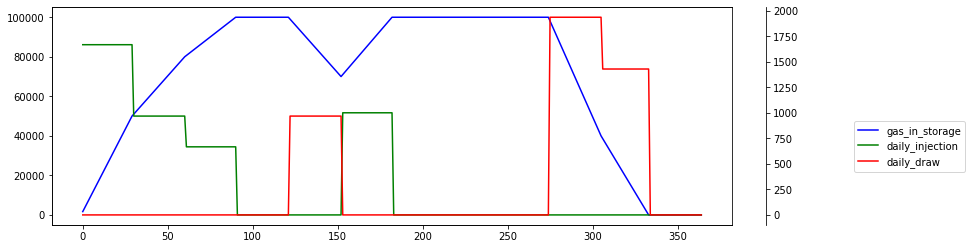In this experiment we're going to use very steep ratchet. A reduction by 75% of max daily injection rate when storage is above 50% of max storage level. Such contract is now worth 23% less then flat ratchet scenario

In :
max_daily_injection3 = pd.DataFrame(data=[(0.0,2000),(0.5,2000),(1.1,500)], columns=['ratchet', 'mdiq'] )
max_daily_withdrawal3 = pd.DataFrame( data=[(0.0,1000),(0.5,1000),(1.1,4000)], columns=['ratchet', 'mdwq'] )
m = build_model( max_daily_injection3, max_daily_withdrawal3 )
solver = pulp.getSolver('PULP_CBC_CMD')
m.solve(solver)
pulp.LpStatus[m.status], pulp.value(m.objective)

Out:
('Optimal', 30400.000000000015)
In :
df_result = pd.DataFrame( data={
'gas_in_storage': map(lambda x: pulp.value(x) , daily_storage),
'daily_injection': map(lambda x: pulp.value(x), daily_injection),
'daily_draw': map(lambda x: pulp.value(x), daily_draw),
})

fig, ax = plt.subplots()
ax3 = ax.twinx()
rspine = ax3.spines['right']
rspine.set_position(('axes', 1.05))
ax3.set_frame_on(True)
ax3.patch.set_visible(False)

<matplotlib.legend.Legend at 0x7f56a57b2ba8>